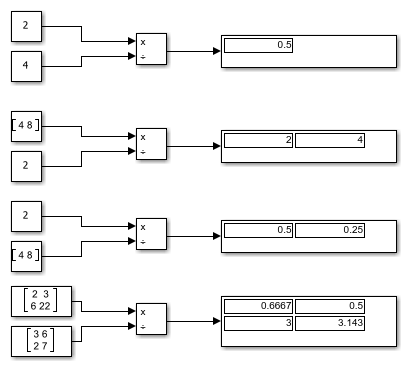Documentation

## Divide Inputs of Different Dimensions Using the Divide Block

This example shows how to perform element-wise `(.*)` division of two inputs using the Divide block. In this example, the Divide block divides two scalars, a vector by a scalar, a scalar by a vector, and two matrices.